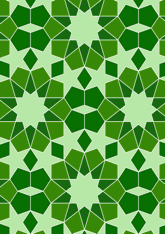# Lee tiling, Figure 31data156/F31

## Geometry

• The symmetry group of the tiling is 2*22 (cmm).
• All the internal angles of the constituent polygons are a multiple of 18°.
• Contains one regular two-pointed star polygon with vertex angle of 36°.
• Contains three regular two-pointed star polygons with vertex angle of 72°.
• Contains two regular pentagons.
• Contains two regular five-pointed star polygons with vertex angle of 36°.
• Contains one regular 10-pointed star polygon with vertex angle of 72°.
• There are two non-regular reflective tiles (including 2 kites).
• The tiling does not satisfy the two-colour condition.
• The tiling is edge-to-edge.
• As drawn, contains about 256 polygons.

## References

Publications referenced:
1. Figure 31 (No colouring in the original, derivied from [shafai] plate 10) of A. J. Lee. Islamic star patterns, Muqarnas vol. 4. pp182-197 Pressmark PP.8.M, 1987. [lee] {Important paper on star patterns} Available on Internet.

## Collections of similar tilings

Patterns using same irregular tiles: 3

v53